• 一、模型建立 根据单摆模型，建立以下模型，如下图： 忽略空气阻力的情况下，当物体离开平衡位置后，物体会受到竖直向下的重力和沿着悬挂线向上方向的拉力的合力作用，使得物体在平衡位置做往返运动。该合力又称为...

一、模型建立
根据单摆模型，建立以下模型，如下图：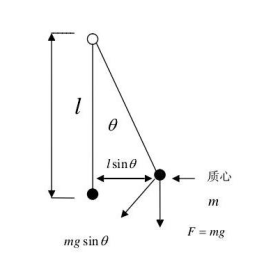忽略空气阻力的情况下，当物体离开平衡位置后，物体会受到竖直向下的重力和沿着悬挂线向上方向的拉力的合力作用，使得物体在平衡位置做往返运动。该合力又称为恢复力，如下：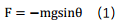其中，悬线与竖直方向夹角为θ，与物体运动方向夹角为 π- θ，F方向与物体运动方向相切，指向平衡位置。
又由极限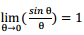，可知，当θ较小时，有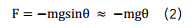实际中，物体除了受到回复力F的作用外，还受到空气阻力F阻的作用，从而最终会静止在平衡位置。而运动中空气阻力的大小与物体运动速度大小成正比，该比例由阻尼系数k表示，而阻力方向与运动速度方向相反。此时物体在运动方向上所受合力如下：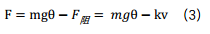因此，对于单摆模型，其保持平衡需耀具备以下条件：

受到与偏移方向相反的回复力的作用     受到与物体运动方向相反的阻尼力的作用对于两轮平衡车，可以简化为倒立摆模型，如下：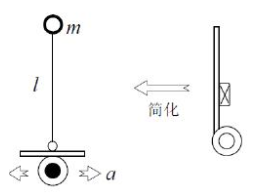而对于倒立摆模型，其受力如下：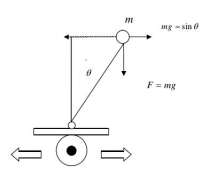如单摆模型，可知物体受到回复力为：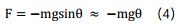但由于此时回复力方向与物体平衡位置方向相反，因此物体反而会加速离开平衡位置。
为了让物体回到平衡位置，即保持与竖直方向平行，可以让倒立摆向与物体偏离方向相反的方向做加速运动，加速度为a。此时倒立摆受力如图：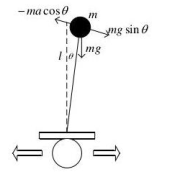倒立摆将受到惯性力：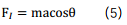不计空气阻力时，倒立摆运动方向上所受合力为：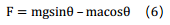当偏移角较小时，有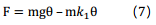其中，取近似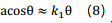当k1>g时，物体在运动方向上受到与回复力方向相反的力。此时，在空气阻力作用下，物体将在平衡位置往返，最终回到平衡位置。而为了让物体尽快回到平衡位置，可以通过外加阻尼力，其大小与物体偏离平衡位置的速度成正比，比例系数为k2，方向相反，则有：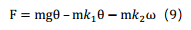因此，可以通过外力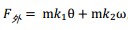，来使小车保持平衡。而外力的来源即是通过控制小车车轮的加速度，使得小车获得相应的惯性力。其中k1会使得小车向物体倾倒的相反方向做加速运动，k1过大过小将导致小车直接偏离品平衡位置的方向而倾倒；k2使得小车能够尽快回到平衡位置，k2过大过小时，都会影响小车回到平衡位置的调节时间。
二、系统分析
第一部分从建立物理模型的角度出发，通过物理定律推导得到物理等式。
但光有理论，没有结合实际也会觉得难以下手，因此第二部分结合实践，从系统的角度出发，结合物理等式对系统的控制做进一步分析。

首先，还是从物理定律出发，得到物理等式。如图，考虑一个可移动小车上的倒立摆模型，其中杆的长度为L，不计杆的质量，杆的末端有一个质量为m的小球，θ(t)该摆偏离垂直位置的角度，g是重力加速度，s(t)是小车相对于某个参考点的位置，a(t)是小车的加速度，x(t)代表由任何扰引起的角加速度。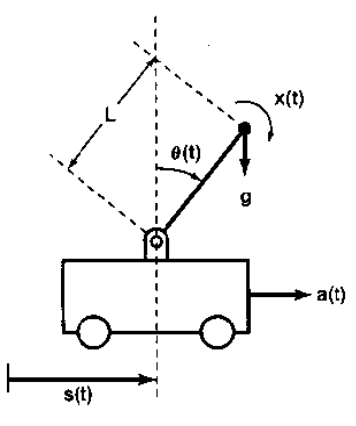对杆上小球受力分析，有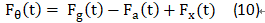此时，Fθ(t)方向为垂直于杆，沿着倾倒方向。
根据运动学知识，有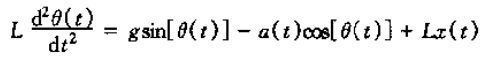（12）
注意，角速度要转为线速度

反馈就是利用一个系统的输出去控制或改变系统的输入。反馈除了能提供一个误差校正的机理而减小系统对扰动以及系统数学模型误差的灵敏度以外，反馈的另一个重要特性在于使一个固有的不稳定系统稳定的能力上。考虑以上倒立摆模型，如果小车保持静止不动的话，倒立摆一定会倒下来。但通过移动小车，让小车根据倒立摆偏离竖直方向的角度来回移动，最终可也让倒立摆达到动态平衡，即与竖直方向角度为0。显然，稳定倒立摆问题也就是设计一个反馈系统。该反馈系统使小车移动以保持倒立摆成垂直状态。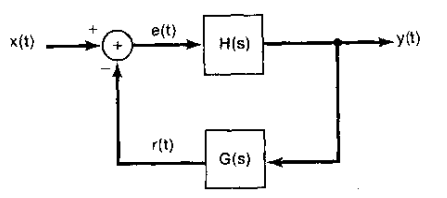对于连续时间LTI反馈系统的一般结构可以通过上图表示，此时系统函数为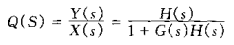（13）

而通过对式（12），假定θ(t)很小，即对于该模型，考虑该模型是在接近垂直位置的时候的动态特性，此时可以做如下近似

sin⁡[ θ(t)  ]≈θ(t)  ,cos⁡[ θ(t)  ]≈1            （14）
当小车静止时，即a(t)=0。结合式（12）和（14），求拉普拉斯变换，可得系统函数为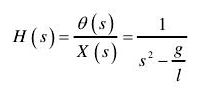（14）
由上式可知，有极点s=g/l 或s=-g/l ，即系统在右半平面有一个极点，意味着该系统是不稳定的。（稳定：系统的单位冲激相应绝对可和。）因此，在不进行反馈控制时，该系统为一个LTI因果不稳定系统。也就是说，在小车静止不动时，任何由x(t)所造成的微小角扰动都将导致偏离垂直方向的角度进一步增大。

为了让小车以适当的方式移动，以摆在垂直位置，设想采用比例反馈，即    a(t) = Kθ(t)   (15)
同样假定θ(t)很小，使得该系统可以近似满足LTI的特性。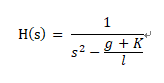（16）
观察发现，此时对于系统函数H(s)，其在s平面的右半平面至少存在一个极点，因此系统依旧不稳定。考虑加入比例加微分反馈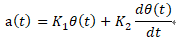，此时该系统函数变为二阶函数，如下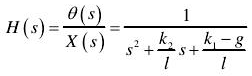（17）
可得系统的两个极点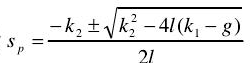。根据奈奎斯特稳定判断依据，要使得系统稳定，则两个极点都必须位于s平面的左半平面，此时有k1>g,k2>0。

综上所述，平衡车控制核心是一个角度PD控制器，有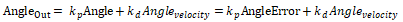（18）
三、改进
实际上，由于结构设计或组装上的不合理，小车物理重心总与竖直方向总存在一个夹角（或者说与地面不是保持垂直关系），小车在这一倾角作用下，在水平方向上受到一重力分量而会进行加速运动，最终倾倒而无法保持平衡。因此，需要在原先角度PD控制的基础上，加入速度PI控制，控制小车的速度。至于为什么是速度PI控制？阅读资料，仍然无法完全理解，其中讲到速度计算中存在着噪声影响，如果对该速度信号做微分计算时，会放大噪声的影响。（或者讲到小车为非最小相位系统，速度调节的时间常数要选取比较大？？不是很理解，如果有更好的见解，也请多多指教哈）
速度PI控制如下：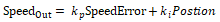（19）

总而言之，小车的控制可以归结为两个PID控制，一个为角度PD控制，一个为速度PI控制，为了保证系统的实时显示，两个PID控制必须放在中断程序中执行，而角度PD控制的频率要比速度PI控制的频率高。（具体还不是很清楚？？）

注：以上部分参考论文和部分网上博客，同时参考《信号系统》第二版（作者：奥本海姆），如有雷同或不当之处，还请指出！！


展开全文arduino
• #include "Wire.h" const uint8_t IMUAddress = 0x68; // AD0 is logic low on the PCB const uint16_t I2C_TIMEOUT = 100; // Used to check for errors in I2C communication int p;...
#include  "Wire.h"
const uint8_t IMUAddress = 0x68; // AD0 is logic low on the PCB
const uint16_t I2C_TIMEOUT = 100; // Used to check for errors in I2C communication
int p;
uint8_t i2cWrite(uint8_t registerAddress, uint8_t data, bool sendStop) {
return i2cWrite(registerAddress, &data, 1, sendStop); // Returns 0 on success
}

uint8_t i2cWrite(uint8_t registerAddress, uint8_t *data, uint8_t length, bool sendStop) {
Wire.write(data, length);
uint8_t rcode = Wire.endTransmission(sendStop); // Returns 0 on success
if (rcode) {
Serial.print(F("i2cWrite failed: "));
Serial.println(rcode);
}
return rcode; // See: http://arduino.cc/en/Reference/WireEndTransmission
}

uint32_t timeOutTimer;
uint8_t rcode = Wire.endTransmission(false); // Don't release the bus
if (rcode) {
Serial.println(rcode);
return rcode; // See: http://arduino.cc/en/Reference/WireEndTransmission
}
Wire.requestFrom(IMUAddress, nbytes, (uint8_t)true); // Send a repeated start and then release the bus after reading
for (uint8_t i = 0; i < nbytes; i++) {
if (Wire.available())
else {
timeOutTimer = micros();
while (((micros() - timeOutTimer) < I2C_TIMEOUT) && !Wire.available());
if (Wire.available())
else {
return 5; // This error value is not already taken by endTransmission
}
}
}
return 0; // Success
}

/******************************************************/

//2560 pin map  引脚定义好即可，然后改变一下PID的几个值（kp，kd，ksp，ksi）即可，剩下的全部都是固定的程序，
//可能小车会有一点重心不在中点的现象，加一下角度值或者减一点即可
//至于每个MPU6050的误差，自己调节一下即可，不是很难
//调试时先将速度环的ksp，ksi=0，调到基本可以站起来，然后可能会出现倒，或者自动跑起来的时候加上速度环
//这时就会很稳定的站起来，然后用小力气的手推不会倒。
int ENA=9;
int ENB=10;
int IN1=7;
int IN2=4;
int IN3=5;
int IN4=6;

int MAS,MBS;
int KEY = 2;

/* IMU Data */
double accX, accY, accZ;
double gyroX, gyroY, gyroZ;
int16_t tempRaw;

double gyroXangle, gyroYangle; // Angle calculate using the gyro only
double compAngleX, compAngleY; // Calculated angle using a complementary filter
double kalAngleX, kalAngleY; // Calculated angle using a Kalman filter
uint8_t i2cData; // Buffer for I2C data
uint32_t timer;
unsigned long lastTime;

/***************************************/
double P = {{ 1, 0 },{ 0, 1 }};
double Pdot ={ 0,0,0,0};
static const double Q_angle=0.001, Q_gyro=0.003, R_angle=0.5,dtt=0.005,C_0 = 1;
double q_bias, angle_err, PCt_0, PCt_1, E, K_0, K_1, t_0, t_1;
double angle,angle_dot,aaxdot,aax;
double position_dot,position_dot_filter,positiono;

/*-------------Encoder---------------*/

#define LF 0
#define RT 1

//The balance PID
float kp,kd,ksp,ksi;

int Lduration,Rduration;
boolean LcoderDir,RcoderDir;
const byte encoder0pinA = 2;
const byte encoder0pinB = 12;//7;
byte encoder0PinALast;
const byte encoder1pinA = 3;
const byte encoder1pinB = 13;//8;
byte encoder1PinALast;

int RotationCoder;
int turn_flag=0;
float move_flag=0;
float right_need = 0, left_need = 0;;

int pwm;
int pwm_R,pwm_L;
float range;
float range_error_all;
float wheel_speed;
float last_wheel;
float error_a=0;

void Kalman_Filter(double angle_m,double gyro_m)
{
angle+=(gyro_m-q_bias) * dtt;
Pdot=Q_angle - P - P;
Pdot=- P;
Pdot=- P;
Pdot=Q_gyro;
P += Pdot * dtt;
P += Pdot * dtt;
P += Pdot * dtt;
P += Pdot * dtt;
angle_err = angle_m - angle;
PCt_0 = C_0 * P;
PCt_1 = C_0 * P;
E = R_angle + C_0 * PCt_0;
K_0 = PCt_0 / E;
K_1 = PCt_1 / E;
t_0 = PCt_0;
t_1 = C_0 * P;
P -= K_0 * t_0;
P -= K_0 * t_1;
P -= K_1 * t_0;
P -= K_1 * t_1;
angle+= K_0 * angle_err;
q_bias += K_1 * angle_err;
angle_dot = gyro_m-q_bias;//也许应该用last_angle-angle
}

void setup() {
Wire.begin();
Serial.begin(9600);
pinMode(KEY, INPUT);
pinMode(IN1, OUTPUT);
pinMode(IN2, OUTPUT);
pinMode(IN3, OUTPUT);
pinMode(IN4, OUTPUT);
pinMode(ENA, OUTPUT);
pinMode(ENB, OUTPUT);
TWBR = ((F_CPU / 400000L) - 16) / 2; // Set I2C frequency to 400kHz

i2cData = 7; // Set the sample rate to 1000Hz - 8kHz/(7+1) = 1000Hz
i2cData = 0x00; // Disable FSYNC and set 260 Hz Acc filtering, 256 Hz Gyro filtering, 8 KHz sampling
i2cData = 0x00; // Set Gyro Full Scale Range to ±250deg/s
i2cData = 0x00; // Set Accelerometer Full Scale Range to ±2g
while (i2cWrite(0x19, i2cData, 4, false)); // Write to all four registers at once
while (i2cWrite(0x6B, 0x01, true)); // PLL with X axis gyroscope reference and disable sleep mode

if (i2cData != 0x68) { // Read "WHO_AM_I" register
while (1);
}
else {
}

delay(20); // Wait for sensor to stabilize

accX = (i2cData << 8) | i2cData;
accY = (i2cData << 8) | i2cData;
accZ = (i2cData << 8) | i2cData;

double roll  = atan2(accX, accZ) * RAD_TO_DEG;
EnCoderInit();
timer = micros();

//The balance PID*************************************************************************
kp= 33;//24.80;43
kd= 2.1 ; //9.66;1.4
ksp= 0;//4.14;8.5
ksi= 0;//0.99; 0.55;2.1
}

void EnCoderInit()
{
//pinMode(8,INPUT);/////////////////////////////////////////////
//pinMode(9,INPUT);
attachInterrupt(LF, LwheelSpeed, RISING);
attachInterrupt(RT, RwheelSpeed, RISING);
}

void pwm_calculate()
{
unsigned long  now = millis();       // 当前时间(ms)
int Time = now - lastTime;
int range_error;
range += (Lduration + Rduration) * 0.5;
range *= 0.9;
range_error = Lduration - Rduration;
range_error_all += range_error;

wheel_speed = range - last_wheel;
//wheel_speed = constrain(wheel_speed,-800,800);
//Serial.println(wheel_speed);
last_wheel = range;
pwm = (angle + 0.825) * kp + angle_dot * kd + range * ksp + wheel_speed * ksi;
if(pwm > 255)pwm = 255;
if(pwm < -255)pwm = -255;

if(turn_flag == 0)
{
pwm_R = pwm + range_error_all;
pwm_L = pwm - range_error_all;
}
else if(turn_flag == 1)     //左转
{
pwm_R = pwm ;  //Direction PID control
pwm_L = pwm + left_need * 68;
range_error_all = 0;     //clean
}
else if(turn_flag == 2)
{
pwm_R = pwm + right_need * 68;  //Direction PID control
pwm_L = pwm ;
range_error_all = 0;     //clean
}

Lduration = 0;//clean
Rduration = 0;
lastTime = now;
}

void PWMD()
{
if(pwm>0)
{
digitalWrite(IN1, HIGH);
digitalWrite(IN2, LOW);
digitalWrite(IN3, LOW);
digitalWrite(IN4, HIGH);
}
else if(pwm<0)
{
digitalWrite(IN1, LOW);
digitalWrite(IN2, HIGH);
digitalWrite(IN3, HIGH);
digitalWrite(IN4, LOW);
}
int PWMr = abs(pwm);
int PWMl = abs(pwm);

analogWrite(ENB, max(PWMl,60)); //PWM调速a==0-255  51
analogWrite(ENA, max(PWMr,60)); //PWM调速a==0-255  54

}

void LwheelSpeed()
{
Lduration++;
else Lduration--;
}

void RwheelSpeed()
{
Rduration--;
else Rduration++;
}

void control()
{
if(Serial.available()){
int val;
switch(val){
case 'w':
if(move_flag<5)move_flag += 0.5;
else  move_flag = 0;
break;
case 's':
if(move_flag<5)move_flag -= 0.5;
else  move_flag = 0;
Serial.println("back");
break;
case 'a':
turn_flag = 1;
left_need = 0.6;
Serial.println("zuo");
break;
case 'd':
turn_flag = 2;
right_need = 0.6;
Serial.println("you");
break;
case 't':
move_flag=0;
turn_flag=0;
right_need = left_need = 0;
Serial.println("stop");
break;
default:
break;
}
}
}

//调试时先将速度环的ksp，ksi=0，调到基本可以站起来，然后可能会出现倒，或者自动跑起来的时候加上速度环
//这时就会很稳定的站起来，然后用小力气的手推不会倒。
void change(){
while (Serial.available() > 0) {
if (ccc == 'P') {
Serial.print("kp:");
Serial.println(kp);
}
else if(ccc == 'D') {
Serial.print("kd:");
Serial.println(kd);
}
else if(ccc == 'K') {
Serial.print("ksp:");
Serial.println(ksp);
}
else if(ccc == 'S') {
Serial.print("ksi:");
Serial.println(ksi);
}
//kp= 200; P //24.80;43
//kd= 2.0 ; D  //9.66;1.4
//ksp= 0; K //4.14;8.5
//ksi= 0;S //0.99; 0.550，2.1
}
}

void loop()
{
//control();
change();
accX = ((i2cData << 8) | i2cData);
//accY = ((i2cData << 8) | i2cData);
accZ = ((i2cData << 8) | i2cData);
//gyroX = (i2cData << 8) | i2cData;
gyroY = (i2cData << 8) | i2cData;
//gyroZ = (i2cData << 8) | i2cData;

////////////////////////////////////////////////////////////////////////////////////////////////////////////////
//Serial.print(accX);Serial.print("  ");
//Serial.print(accZ);Serial.print("  ");
//Serial.println(gyroY);
////////////////////////////////////////////////////////////////////////////////////////////////////////////////

double dt = (double)(micros() - timer) / 1000000; // Calculate delta time
timer = micros();

double roll  = atan2(accX, accZ) * RAD_TO_DEG - move_flag;

//double gyroXrate = gyroX / 131.0; // Convert to deg/s
double gyroYrate = -gyroY / 131.0; // Convert to deg/s

//gyroXangle += gyroXrate * dt; // Calculate gyro angle without any filter
//gyroYangle += gyroYrate * dt;

Kalman_Filter(roll,gyroYrate);

if(abs(angle)<35){
//Serial.println(angle);    while (Serial.available() > 0) {   // 串口收到字符数大于零。
pwm_calculate();
PWMD();
}
else{
analogWrite(ENB, 0); //PWM调速a==0-255
analogWrite(ENA, 0); //PWM调速a==0-255
}
delay(2);
}

展开全文• Aeduino自平衡小车Aeduino自平衡小车Aeduino自平衡小车Aeduino自平衡小车Arduino
• mpu6050得到的角度值有些值的偏差较大，为了使平衡小车更加稳定，需要对获得的角度进行优化，使用 卡尔曼滤波，代码如下： #include <MPU6050.h> //MPU6050的库文件 #include <Wire.h> //IIC通讯库文件...
对mpu6050获得的X轴角度和角速度进行卡尔曼滤波
mpu6050得到的角度值有些值的偏差较大，为了使平衡小车更加稳定，需要对获得的角度进行优化，使用 卡尔曼滤波，代码如下：

#include <MPU6050.h>      //MPU6050的库文件
#include <Wire.h>        //IIC通讯库文件

MPU6050 mpu6050;     //实例化一个MPU6050对象，对象名称为mpu6050
int16_t ax, ay, az, gx, gy, gz;     //定义三轴加速度，三轴陀螺仪的变量
float Angle;
float Gyro_x,Gyro_y,Gyro_z;  //用于陀螺仪算出的各轴角速度

///////////////////////Kalman_Filter////////////////////////////
float Q_angle = 0.001;  //陀螺仪噪声的协方差
float Q_gyro = 0.1;    //陀螺仪漂移噪声的协方差
float R_angle = 0.5;    //加速度计的协方差
char C_0 = 1;
float dt = 0.005; //dt的取值为滤波器采样时间
float K1 = 0.05; // 含有卡尔曼增益的函数，用于计算最优估计值的偏差
float K_0,K_1,t_0,t_1;
float angle_err;
float q_bias;    //陀螺仪漂移

float accelz = 0;
float angle;
float angle_speed;

float Pdot = { 0, 0, 0, 0};
float P = {{ 1, 0 }, { 0, 1 }};
float  PCt_0, PCt_1, E;
//////////////////////Kalman_Filter/////////////////////////

void setup()
{
// 加入I2C总线
Wire.begin();                            //加入 I2C 总线序列
Serial.begin(9600);                       //开启串口，设置波特率为9600
delay(1500);
mpu6050.initialize();                       //初始化MPU6050
delay(2);
}

void loop()
{
Serial.print("Angle = ");
Serial.print(Angle);
Serial.print("     K_angle = ");
Serial.println(angle);

Serial.print("Gyro_x = ");
Serial.print(Gyro_x);
Serial.print("    K_Gyro_x = ");
Serial.println(angle_speed);

mpu6050.getMotion6(&ax, &ay, &az, &gx, &gy, &gz);     //IIC获取MPU6050六轴数据 ax ay az gx gy gz
angle_calculate(ax, ay, az, gx, gy, gz, dt, Q_angle, Q_gyro, R_angle, C_0, K1);      //获取angle 角度和卡曼滤波
}

/////////////////////////////倾角计算///////////////////////
void angle_calculate(int16_t ax,int16_t ay,int16_t az,int16_t gx,int16_t gy,int16_t gz,float dt,float Q_angle,float Q_gyro,float R_angle,float C_0,float K1)
{
Angle = atan2(ay , az) * (180/ PI);           //弧度转角度计算公式,负号为方向处理
Gyro_x = gx / 131;              //陀螺仪计算的X轴角速度，负号为方向处理
Kalman_Filter(Angle, Gyro_x);            //卡曼滤波
}
////////////////////////////////////////////////////////////////

///////////////////////////////KalmanFilter/////////////////////
void Kalman_Filter(double angle_m, double gyro_m)
{
angle += (gyro_m - q_bias) * dt;          //先验估计
angle_err = angle_m - angle;

Pdot = Q_angle - P - P;    //先验估计误差协方差的微分
Pdot = - P;
Pdot = - P;
Pdot = Q_gyro;

P += Pdot * dt;    //先验估计误差协方差微分的积分
P += Pdot * dt;
P += Pdot * dt;
P += Pdot * dt;

//矩阵乘法的中间变量
PCt_0 = C_0 * P;
PCt_1 = C_0 * P;
//分母
E = R_angle + C_0 * PCt_0;
//增益值
K_0 = PCt_0 / E;
K_1 = PCt_1 / E;

t_0 = PCt_0;  //矩阵乘法的中间变量
t_1 = C_0 * P;

P -= K_0 * t_0;    //后验估计误差协方差
P -= K_0 * t_1;
P -= K_1 * t_0;
P -= K_1 * t_1;

q_bias += K_1 * angle_err;    //后验估计
angle_speed = gyro_m - q_bias;   //输出值的微分，得出最优角速度
angle += K_0 * angle_err; ////后验估计，得出最优角度
}




展开全文arduino
• arduino 霍尔编码器测速
MsTime2，霍尔编码器测速
100毫秒触发一次中断，打印出这100毫秒内，霍尔编码器测到的脉冲数。
代码如下：
#include <MsTimer2.h>

//TB6612引脚定义
const int right_R1=8;
const int right_R2=12;
const int PWM_R=10;
const int left_L1=7;
const int left_L2=6;
const int PWM_L=9;

const int PinA_left = 5;        //定义检测左电机脉冲的引脚为D5
const int PinA_right = 4;       //定义检测右电机脉冲的引脚为D4

int times=0,newtime=0,d_time=100;   //时间，最新的时间，时间间隔
int valA=0,valB=0,flagA=0,flagB=0;   //变量valA和valB用于计算脉冲数

void setup()
{
Serial.begin(9600);

pinMode(right_R1,OUTPUT);     //TB6612的引脚都设置为输出
pinMode(right_R2,OUTPUT);
pinMode(PWM_R,OUTPUT);
pinMode(left_L1,OUTPUT);
pinMode(left_L2,OUTPUT);
pinMode(PWM_L,OUTPUT);

pinMode(PinA_left,INPUT);      //设置检测脉冲的引脚为输入状态
pinMode(PinA_right,INPUT);

MsTimer2::set(100, inter); // 100ms 触发一次中断，调用函数inter()
MsTimer2::start();    //开启中断
}

void loop()
{
//两电机都正转
digitalWrite(right_R1,HIGH);
digitalWrite(right_R2,LOW);
digitalWrite(left_L1,HIGH);
digitalWrite(left_L2,LOW);
analogWrite(PWM_R,100);    //写入PWM值0~255（速度）
analogWrite(PWM_L,200);

{
valA++;
flagA=1;
}
{
valA++;
flagA=0;
}

{
valB++;
flagB=1;
}
{
valB++;
flagB=0;
}

}

//中断函数
void inter()
{
sei();    //允许全局中断
Serial.print("valA = ");     //在串口监视器上打印出脉冲值
Serial.println(valA);
Serial.print("valB = ");
Serial.println(valB);
valA = valB = 0;         //清0
}



展开全文• 自平衡小车arduino主控+mpu6050+卡尔曼滤波+PID
• 完成后能基本保持平衡，但太大力去推容易倒 平衡原理  通过负反馈实现平衡，车体会在轮子滚动的方向上发生倾斜。控制轮子转动，抵消在一个维度上倾斜的趋势便可以保持车体平衡了。根据这个原理，通过...

完成样子
因为只是学习验证，没用电烙铁，只用了面包板来连接各个组件，中间用扎带固定（不稳定）
完成后能基本保持平衡，但太大力去推容易倒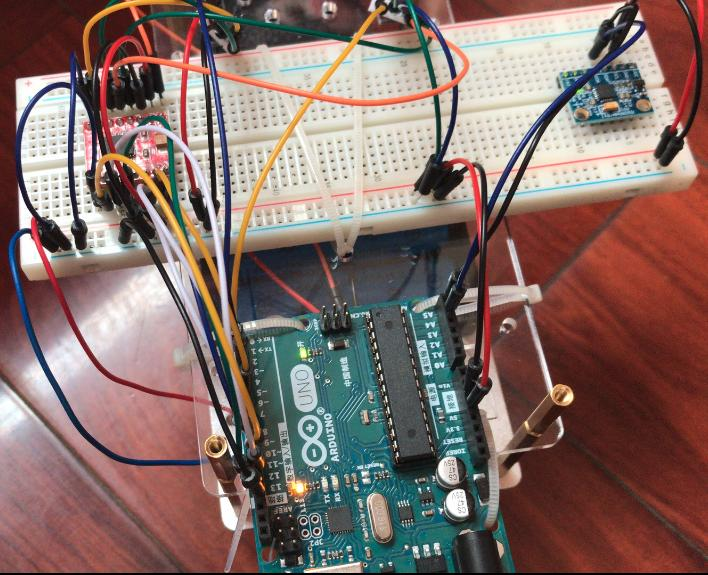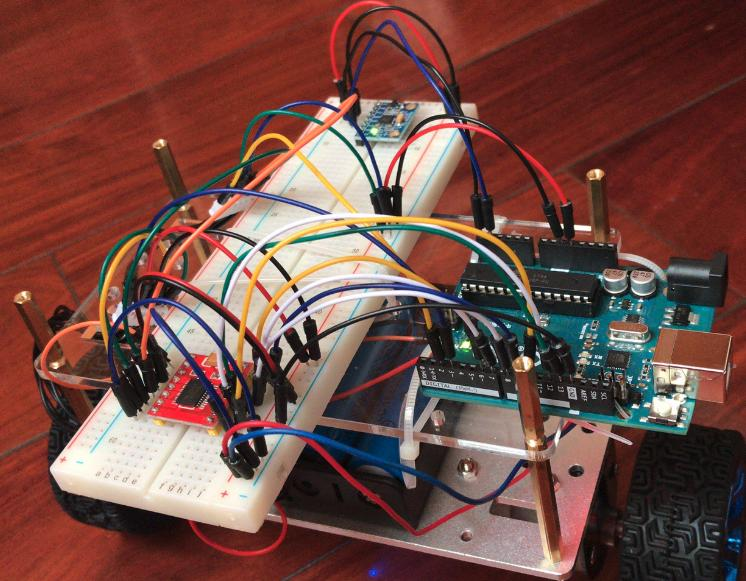平衡原理
通过负反馈实现平衡，车体会在轮子滚动的方向上发生倾斜。控制轮子转动，抵消在一个维度上倾斜的趋势便可以保持车体平衡了。根据这个原理，通过测量小车的倾角和倾角速度控制小车车轮的加速度来消除小车的倾角。因此,小车倾角以及倾角速度的测量成为控制小车直立的关键，因此使用了测量倾角和倾角速度的集成传感器陀螺仪-MPU6050。

实现细节
这是用fritzing画的Arduino与TB6612FNG电机驱动模块 和 MPU6050 模块之间的电路连接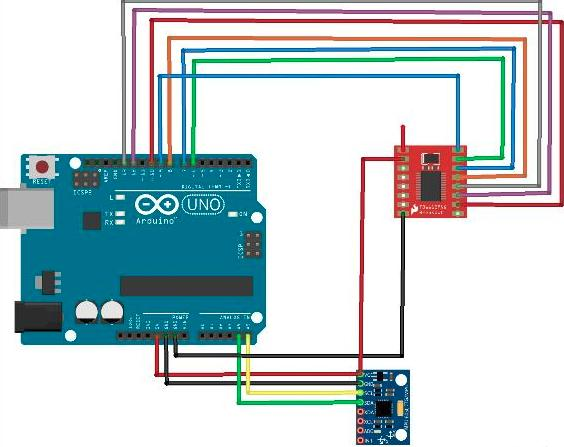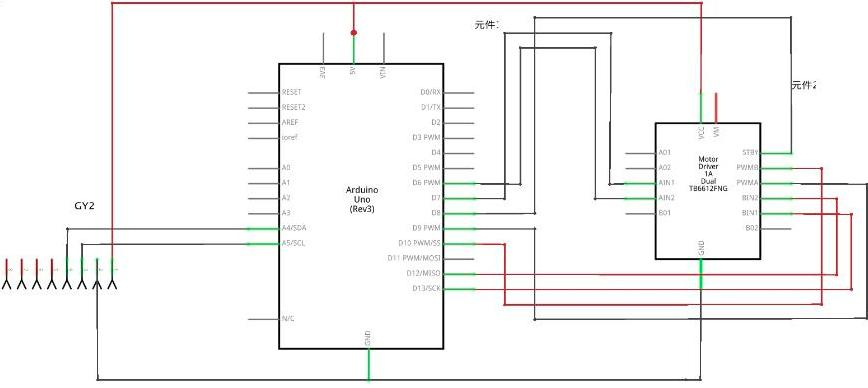供电部分
电机驱动模块TB6612FNG的VM必须要接上12v不然电机供电不足，这里用了3个18650供电，通过LM2596S变压为5v给Arduino供电

电机部分
使用的是GB37-520电机，减速比为 1:30，带霍尔传感器测速码盘
1--- --电机电源线 AO1
2--- --电机电源线 AO2
3--- --传感器信号线 负电 GND
4--- --传感器 正电 5V VCC
5--- --传感器信号线 B 相
6--- --传感器信号线 A 相


展开全文Arduino
•   平衡车 Arduino实战全攻略，15个创客喜爱的Arduino实例制作项目arduino
• 基于arduino2560平衡车程序，绝对真实有用，还带有其他功能，比如语音模块，全贡献出来了
• 在受到Segway的RYNO Motors电动独轮摩托和其他自平衡滑板的启发后，我一直想要制作一个类似的东西。基于这一点，我决定使用Arduino开发板制作一个自平衡机器人。通过这种方式，我将能够掌握所有这些踏板背后的...
• 摘 要: 两轮自平衡小车被各种竞赛所喜爱，在很多大型比赛中都有涉及此方向的题目。阐述了基于飞思卡尔系列单片机为核心控制器，配合陀螺仪、角度传感器实现两轮小车的自平衡。着重介绍了两轮平 衡小车的结构、平衡...自平衡小车
• 1.硬件电路 从左往右，依次是MPU6050陀螺仪模块，Promin 开发板，DRV8833电机驱动，两块升压板，将18650电池电压升高至5V。减小干扰，驱动电路与控制电路分离，所以用了两块升压板。依赖的库在代码头部说明里，代码...arduino
• 两轮自平衡小车（卡尔曼滤波代码+PID调试文档），包含详细的代码，stm32为控制器。
• 如何在家自己动手做一个arduino平衡车？简单的源代码分享，优酷有我的视频！
• 使用mpu6050的x轴的角度来作为自平衡小车的平衡角度。 故求x轴角度，代码如下： #include <MPU6050.h> //MPU6050的库文件 #include <Wire.h> //IIC通讯库文件 MPU6050 mpu6050; //实例化一个MPU6050...
• 为了学习PID算法和简单的惯性导航，开始学习制作平衡小车。 文章分为三部分，第一部分，读取MPU6050的原始输出，通过滤波算法获取传感器角度 。 第二部分，小车的一些简单建模和数学计算公式。第三部分，小车的PID...
• （1）主控板 -- Arduino UNO R3技术规格工作电压：5V输入电压：接上USB时无须外部供电或外部7V~12V DC输入输出电压：5V DC输出和3.3V DC输出 和外部电源输入微处理器：ATmega328Bootloader：Arduino Uno时钟频率：16...arduino 两轮平衡车
• 平衡小车之家两轮平衡小车程序,包括arduino和STM32的程序及原理图,硬件参考资料,驱动和调试软件,开发环境软件和库文件,测速码盘电机详细讲解资料等,可以说是非常全面的
• 首先介绍一下mpu6050，反正就是挺好用的，自己百度吧，写多了看也看不懂。 重要的地方讲讲。首先是mpu6050配置 void set_gyro_registers(){ //Setup the MPU-6050 if(eeprom_data == 1){ ...
• 所以我们就用arduino+普通的香蕉电机+驱动模块+超声波做一个两轮平衡车。 首先是硬件组装，这部分很简单，就是拿个电路板把电机和轮子装好，然后在上方布局nano板，电池，最好放置的时候就让重...
...